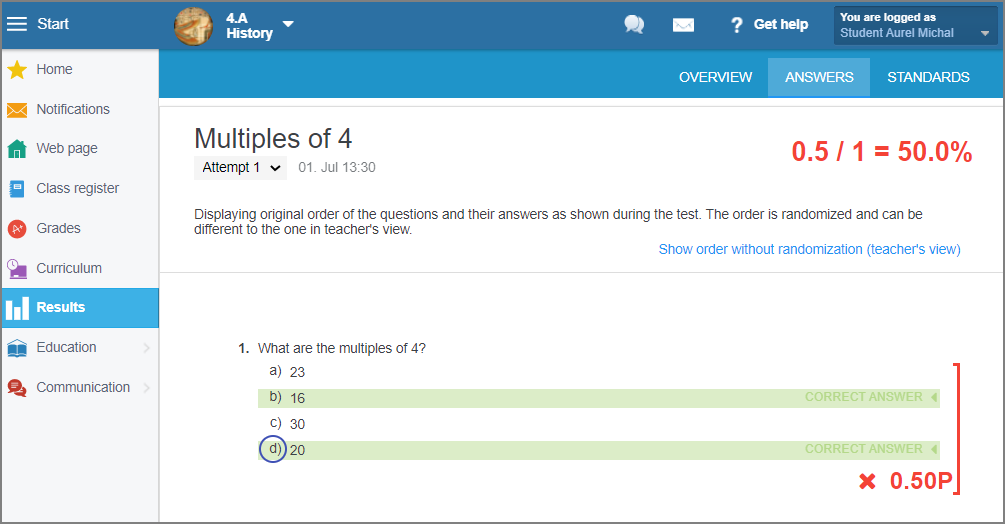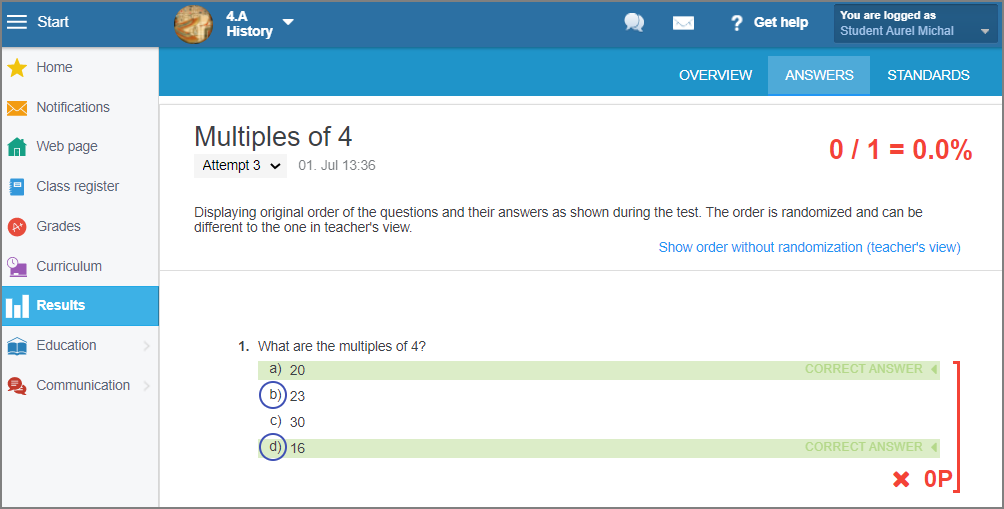EN# ABCD question with more than one correct answer

After clicking on one of the answers in the ABCD question, a "Multiple choice options" box appears on the right side of the screen. Select the "1 or more are correct" option. You will be able to tag multiple answers as correct.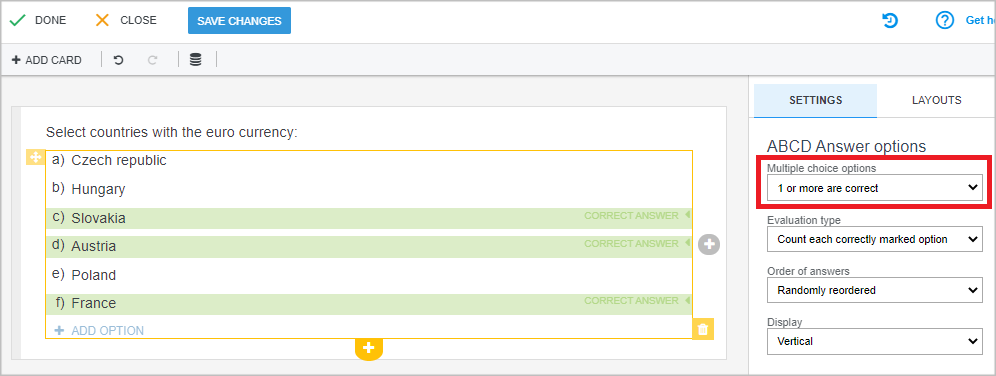Evaluating questions with more than one correct answer:

There are two evaluation options:

a) Count each correctly marked option
b) The number of correct answers minus the number of incorrect answers marked, with 0 as minimum score.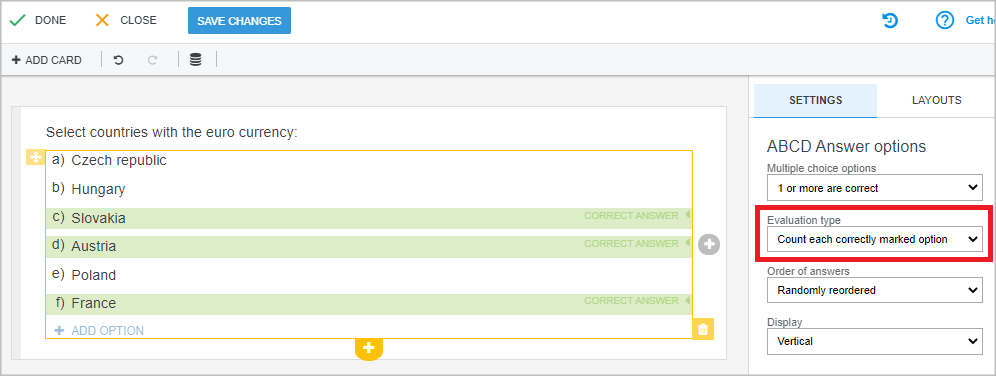Following is the explanation of evaluating in both of the options.

a) Count each correctly marked option:

Each answer option is either correct or incorrect. The student tags the answers they consider as correct, i.e. leaves the incorrect answers untagged (as if tagged incorrect).

That is how the system understands tagging answers.

Thus the result is the number of correct tags compared to the total number of options.
The same applies to questions with 0 correct answers.

Example: A question has 6 answer options. Three of them are correct, three are incorrect. If a student tags 2 of the answers as correct, the untagged answers are considered to be incorrect by the student. The student made a mistake in one instance, when they considered a correct answer as incorrect. It means, the student was right in 5 cases out of 6. The student was awarded 83.3% for the question.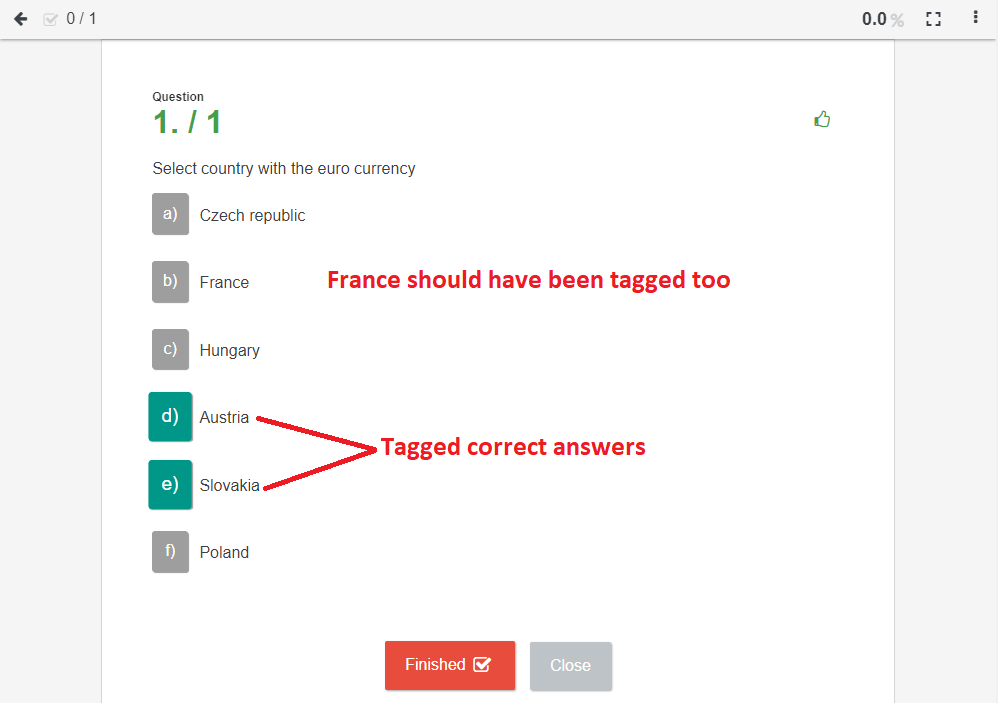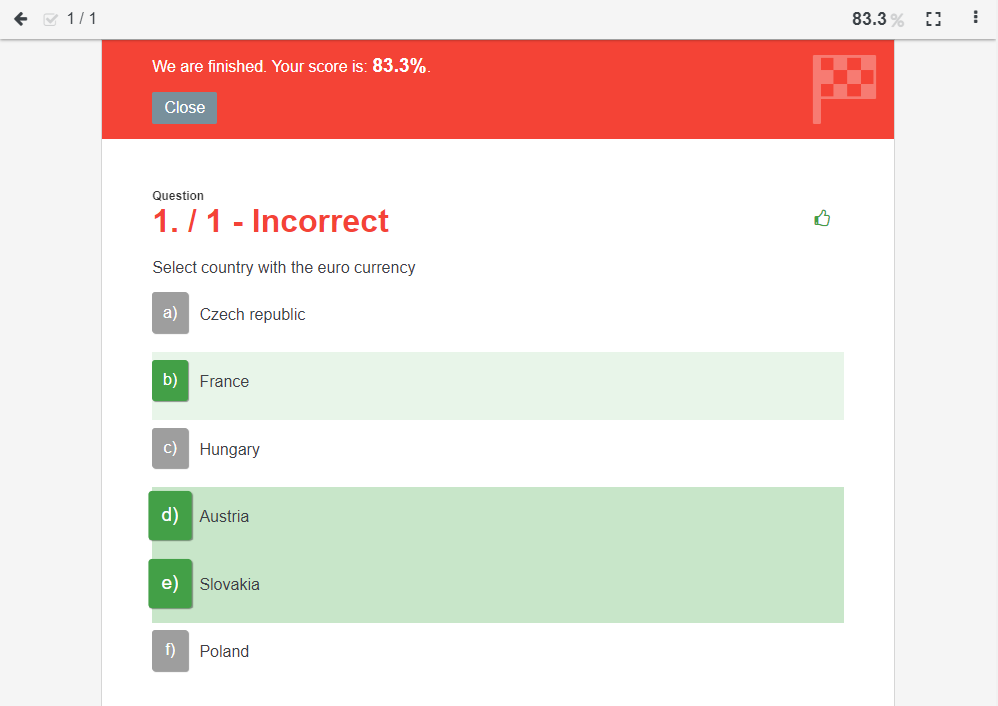b) The number of correct answers minus the number of incorrect answers marked, with 0 as minimum score.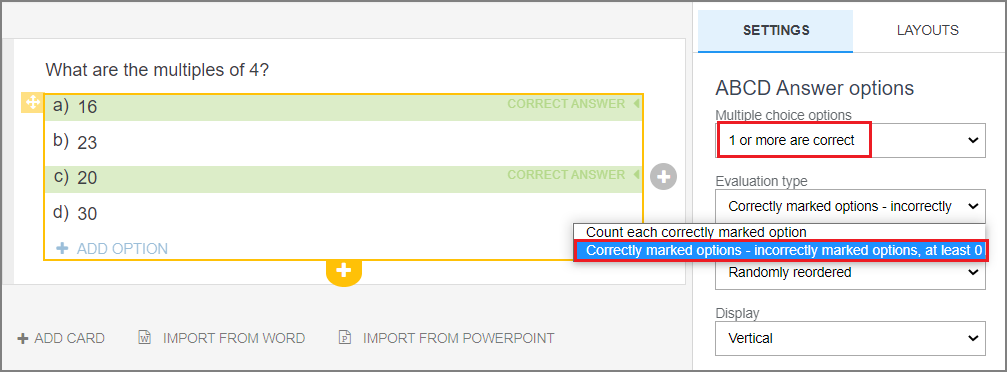The system evaluates the ticked answers only.
If there is a question with two correct answers and the student tags only one of them, it is 50%.